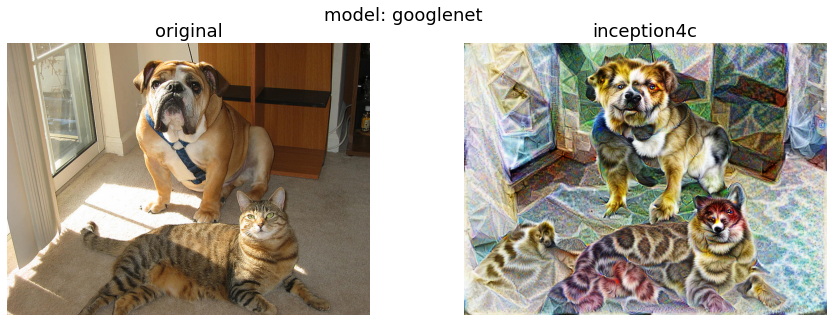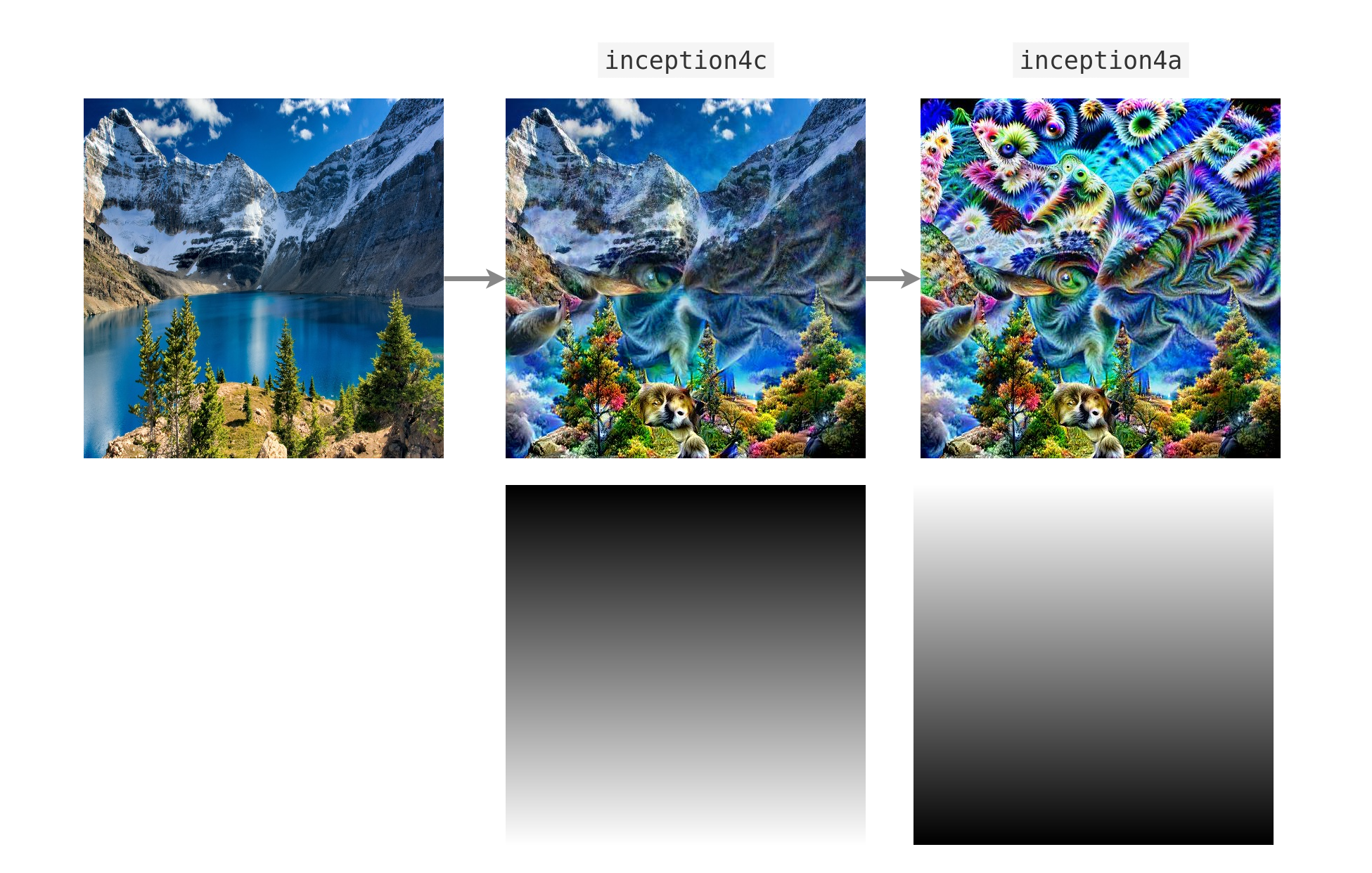Showing:

0

190

3mos ago

4

6

# Torch-Dreams

Making neural networks more interpretable, for research and art.``````pip install torch-dreams
``````

## Minimal example

Make sure you also check out the quick start colab notebook

``````import matplotlib.pyplot as plt
import torchvision.models as models
from torch_dreams.dreamer import dreamer

model = models.inception_v3(pretrained=True)
dreamy_boi = dreamer(model, device = 'cuda')

image_param = dreamy_boi.render(
layers = [model.Mixed_5b],
)

plt.imshow(image_param)
plt.show()
``````

## Not so minimal example

``````model = models.inception_v3(pretrained=True)
dreamy_boi = dreamer(model, device = 'cuda', quiet = False)

image_param = dreamy_boi.render(
layers = [model.Mixed_5b],
width = 256,
height = 256,
iters = 150,
lr = 9e-3,
rotate_degrees = 15,
scale_max = 1.2,
scale_min =  0.5,
translate_x = 0.2,
translate_y = 0.2,
custom_func = None,
weight_decay = 1e-2,
)

plt.imshow(image_param)
plt.show()
``````

## Visualizing individual channels with `custom_func`

``````model = models.inception_v3(pretrained=True)
dreamy_boi = dreamer(model, device = 'cuda')

layers_to_use = [model.Mixed_6b.branch1x1.conv]

def make_custom_func(layer_number = 0, channel_number= 0):
def custom_func(layer_outputs):
loss = layer_outputs[layer_number][channel_number].mean()
return -loss
return custom_func

my_custom_func = make_custom_func(layer_number= 0, channel_number = 119)

image_param = dreamy_boi.render(
layers = layers_to_use,
custom_func = my_custom_func,
)
plt.imshow(image_param)
plt.show()
``````

## Caricatures

Caricatures create a new image that has a similar but more extreme activation pattern to the input image at a given layer (or multiple layers at a time). It's inspired from this issueIn this case, let's use googlenet

``````model = models.googlenet(pretrained = True)
dreamy_boi = dreamer(model = model, quiet= False, device= 'cuda')

image_param = dreamy_boi.caricature(
input_tensor = image_tensor,
layers = [model.inception4c],   ## feel free to append more layers for more interesting caricatures
power= 1.2,                     ## higher -> more "exaggerated" features
)

plt.imshow(image_param)
plt.show()
``````

## Visualize features from multiple models simultaneously

First, let's pick 2 models and specify which layers we'd want to work with

``````from torch_dreams.model_bunch import ModelBunch

bunch = ModelBunch(
model_dict = {
'inception': models.inception_v3(pretrained=True).eval(),
'resnet':    models.resnet18(pretrained= True).eval()
}
)

layers_to_use = [
bunch.model_dict['inception'].Mixed_6a,
bunch.model_dict['resnet'].layer2.conv1
]

dreamy_boi = dreamer(model = bunch, quiet= False, device= 'cuda')
``````

Then define a `custom_func` which determines which exact activations of the models we have to optimize

``````def custom_func(layer_outputs):
loss =   layer_outputs.mean()*2.0 + layer_outputs.mean()
return -loss
``````

Run the optimization

``````image_param = dreamy_boi.render(
layers = layers_to_use,
custom_func= custom_func,
iters= 100
)

plt.imshow(image_param)
plt.show()
``````

## Using custom transforms:

``````import torchvision.transforms as transforms

model = models.inception_v3(pretrained=True)
dreamy_boi = dreamer(model,  device = 'cuda', quiet =  False)

my_transforms = transforms.Compose([
transforms.RandomAffine(degrees = 10, translate = (0.5,0.5)),
transforms.RandomHorizontalFlip(p = 0.3)
])

dreamy_boi.set_custom_transforms(transforms = my_transforms)

image_param = dreamy_boi.render(
layers = [model.Mixed_5b],
)

plt.imshow(image_param)
plt.show()
``````

## You can also use outputs of one `render()` as the input of another to create feedback loops.

``````import matplotlib.pyplot as plt
import torchvision.models as models
from torch_dreams.dreamer import dreamer

model = models.inception_v3(pretrained=True)
dreamy_boi = dreamer(model,  device = 'cuda', quiet =  False)

image_param = dreamy_boi.render(
layers = [model.Mixed_6c],
)

image_param = dreamy_boi.render(
image_parameter= image_param,
layers = [model.Mixed_5b],
iters = 20
)

plt.imshow(image_param)
plt.show()
``````

## Using custom images

Note that you might have to use smaller values for certain hyperparameters like `lr` and `grad_clip`.

``````from torch_dreams.custom_image_param import custom_image_param
param = custom_image_param(image = 'images/sample_small.jpg', device= 'cuda')  ## image could either be a filename or a torch.tensor of shape NCHW

image_param = dreamy_boi.render(
image_parameter= param,
layers = [model.Mixed_6c],
lr = 2e-4,
weight_decay= 1e-1,
iters = 120
)
``````

## Working on models with different image normalizations

`torch-dreams` generally works with models trained on images normalized with imagenet `mean` and `std`, but that can be easily overriden to support any other normalization. For example, if you have a model with `mean = [0.5, 0.5, 0.5]` and `std = [0.5, 0.5, 0.5]`:

``````t = torchvision.transforms.Normalize(
mean = [0.5, 0.5, 0.5],
std =  [0.5, 0.5, 0.5]
)

dreamy_boi.set_custom_normalization(normalization_transform = t) ## normalization_transform could be any instance of torch.nn.Module
``````

Can be used to optimize only certain parts of the image using a mask whose values are clipped between `[0,1]`.Here's an example with a vertical gradient

``````from torch_dreams.masked_image_param import masked_image_param

for i in range(0, 512, 1):  ## vertical gradient

image= 'images/sample_small.jpg',  ## optional
device = 'cuda'
)

param = dreamy_boi.render(
layers = [model.inception4c],
image_parameter= param,
lr = 1e-4,
weight_decay= 1e-1,
iters= 200,
)

``````

It's also possible to update the mask on the fly with `param.update_mask(some_mask)`

``````

param = dreamy_boi.render(
layers = [model.inception4a],
image_parameter= param,
lr = 1e-4,
weight_decay= 1e-1,
iters= 200,
)

``````

## Other conveniences

The following methods are handy for an `auto_image_param` instance:

1. Saving outputs as images:
``````image_param.save('output.jpg')
``````
1. Torch Tensor of dimensions `(height, width, color_channels)`
``````torch_image = image_param.to_hwc_tensor(device = 'cpu')
``````
1. Torch Tensor of dimensions `(color_channels, height, width)`
``````torch_image_chw = image_param.to_chw_tensor(device = 'cpu')
``````
1. Displaying outputs on matplotlib.
``````plt.imshow(image_param)
plt.show()
``````
1. For instances of `custom_image_param`, you can set any NCHW tensor as the image parameter:
``````image_tensor = image_param.to_nchw_tensor()

## do some stuff with image_tensor
t = transforms.Compose([
transforms.RandomRotation(5)
])
transformed_image_tensor = t(image_tensor)

image_param.set_param(tensor = transformed_image_tensor)
``````

## Args for `render()`

• `layers` (`iterable`): List of the layers of model(s)'s layers to work on. `[model.layer1, model.layer2...]`

• `image_parameter` (`auto_image_param`, optional): Instance of `torch_dreams.auto_image_param.auto_image_param`

• `width` (`int`, optional): Width of image to be optimized

• `height` (`int`, optional): Height of image to be optimized

• `iters` (`int`, optional): Number of iterations, higher -> stronger visualization

• `lr` (`float`, optional): Learning rate

• `rotate_degrees` (`int`, optional): Max rotation in default transforms

• `scale_max` (`float`, optional): Max image size factor. Defaults to 1.1.

• `scale_min` (`float`, optional): Minimum image size factor. Defaults to 0.5.

• `translate_x` (`float`, optional): Maximum translation factor in x direction

• `translate_y` (`float`, optional): Maximum translation factor in y direction

• `custom_func` (`function`, optional): Can be used to define custom optimiziation conditions to `render()`. Defaults to None.

• `weight_decay` (`float`, optional): Weight decay for default optimizer. Helps prevent high frequency noise. Defaults to 0.

• `grad_clip` (`float`, optional): Maximum value of the norm of gradient. Defaults to 1.

## Args for `dreamer.__init__()`

• `model` (`nn.Module` or `torch_dreams.model_bunch.Modelbunch`): Almost any PyTorch model which was trained on imagenet `mean` and `std`, and supports variable sized images as inputs. You can pass multiple models into this argument as a `torch_dreams.model_bunch.Modelbunch` instance.
• `quiet` (`bool`): Set to `True` if you want to disable any progress bars
• `device` (`str`): `cuda` or `cpu` depending on your runtime

## Rate & Review

Great Documentation0
Easy to Use0
Performant0
Highly Customizable0
Bleeding Edge0
Responsive Maintainers0
Poor Documentation0
Hard to Use0
Slow0
Buggy0
Abandoned0
Unwelcoming Community0
100